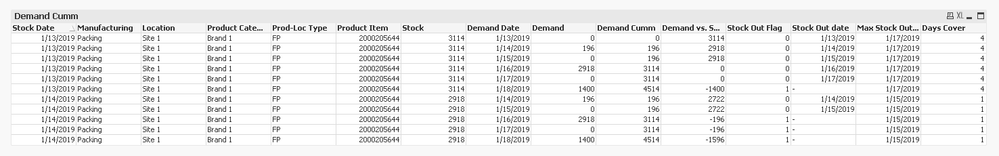# QlikView Creating Analytics

Discussion Board for collaboration related to Creating Analytics for QlikView.

Announcements
Modernize Your QlikView Deployment webinar, Nov. 3rd. REGISTER
cancel
Showing results for
Did you mean:
HighlightedCreator

## Days Cover Logic Calculation in QlikView

Dear All, We have a requirement to create a Days Cover formula. Attached the sample file. Could anyone help me on the calculation? Thanks, Srini.

Labels (5)

• ### ROLLING SUM

5 Replies
HighlightedMVP

Are the first 13 rows in the Excel how your data looks like? If it is, why can't you just do this If(Demand Date = Stock Date, Days Cover)? I mean I am not sure what the complication is?

HighlightedCreator

Sorry for the confusion.

The column from A to I is available.

But the other columns need to be derived and the final output as per row 18 should be created.

Hope it is clear now.

Attached the updated file as per the comments.

Thanks,
Srini.

HighlightedMVP

These needed to be calculated in the script or on the front end of the app? Also, you need to see just the two rows with Days Cover  = 4 or do you need all rows to say Days Cover = 4 for Stock Date = 1/13/2019?

HighlightedCreator
I want to display for Stock Date = 1/13/2019, Days cover as 4.
I would like to know whether I will be able to calculation in Front end or in Back end?
Which is the correct approach?
Any steps to achieve in Front end or Back end?

Thanks,
Srini.
HighlightedMVP

For Stock Date 1/14/2019, I am getting Days cover of 1 because my demand calculation is 196 for Demand date 1/14 and 1/15, why do you have 0?Leaving that issue aside, I did it in the front end and got thisExpressions

Demand Cumm

`=Aggr(RangeSum(Above(Sum(Demand), 0, RowNo())), [Stock Date], [Demand Date])`

Demand vs. Stock

`=Stock - Aggr(RangeSum(Above(Sum(Demand), 0, RowNo())), [Stock Date], [Demand Date])`

Stock Out Flag

`=If(Stock - Aggr(RangeSum(Above(Sum(Demand), 0, RowNo())), [Stock Date], [Demand Date]) < 0, 1, 0)`

Stock Out date

`=If(Stock - Aggr(RangeSum(Above(Sum(Demand), 0, RowNo())), [Stock Date], [Demand Date]) < 0, Null(), [Demand Date])`

Max Stock Out Date

`=Max(TOTAL <[Stock Date]> Aggr(If(Stock - Aggr(RangeSum(Above(Sum(Demand), 0, RowNo())), [Stock Date], [Demand Date]) < 0, Null(), [Demand Date]), [Stock Date], [Demand Date]))`

Days Cover

`=Max(TOTAL <[Stock Date]> Aggr(If(Stock - Aggr(RangeSum(Above(Sum(Demand), 0, RowNo())), [Stock Date], [Demand Date]) < 0, Null(), [Demand Date]), [Stock Date], [Demand Date])) - [Stock Date]`